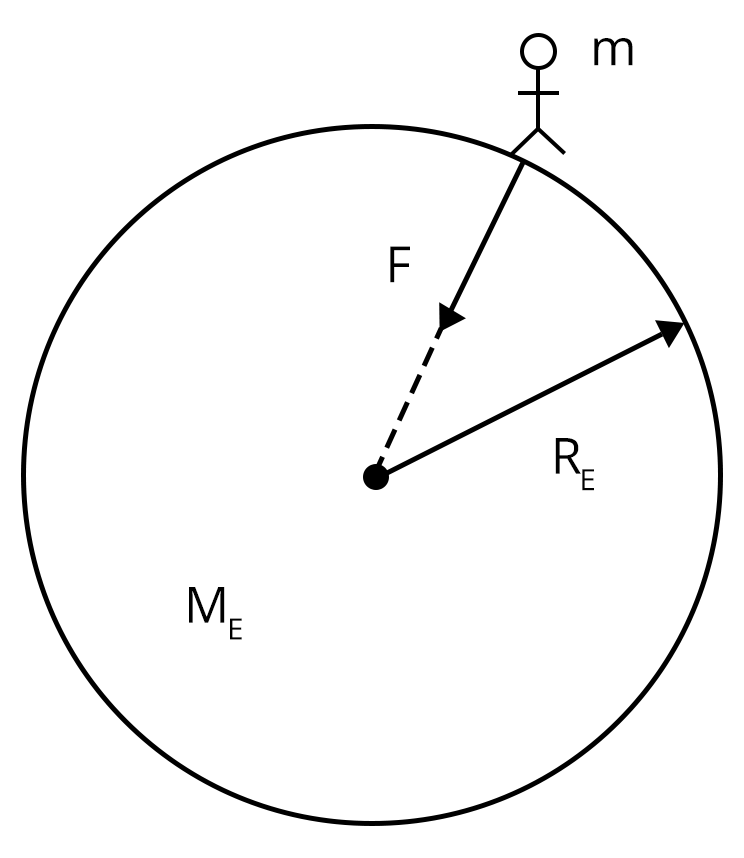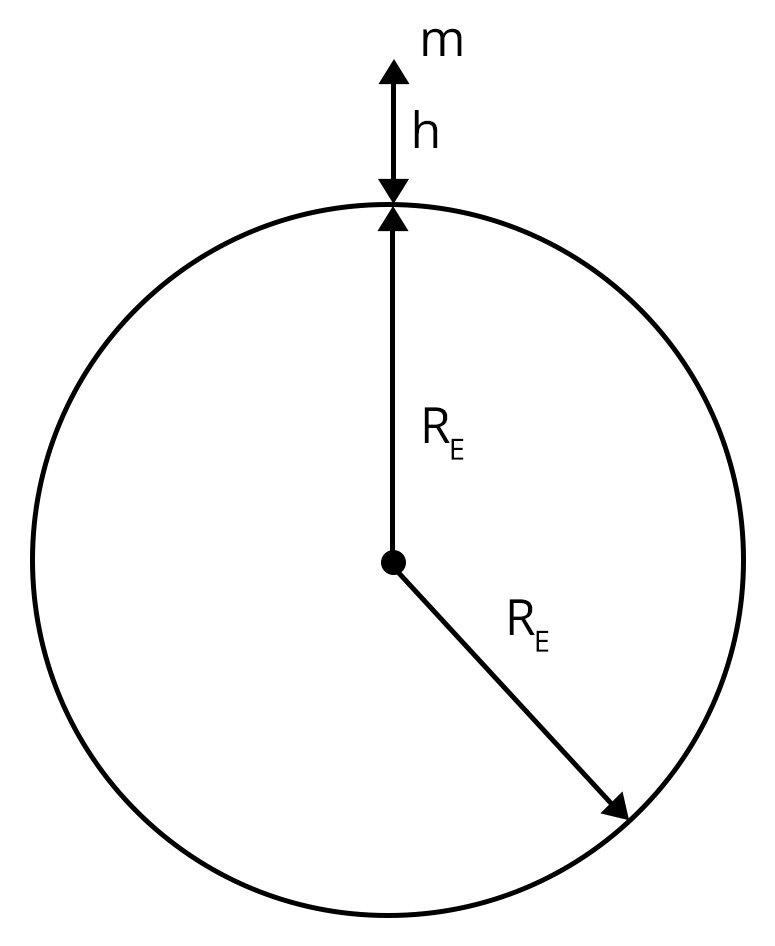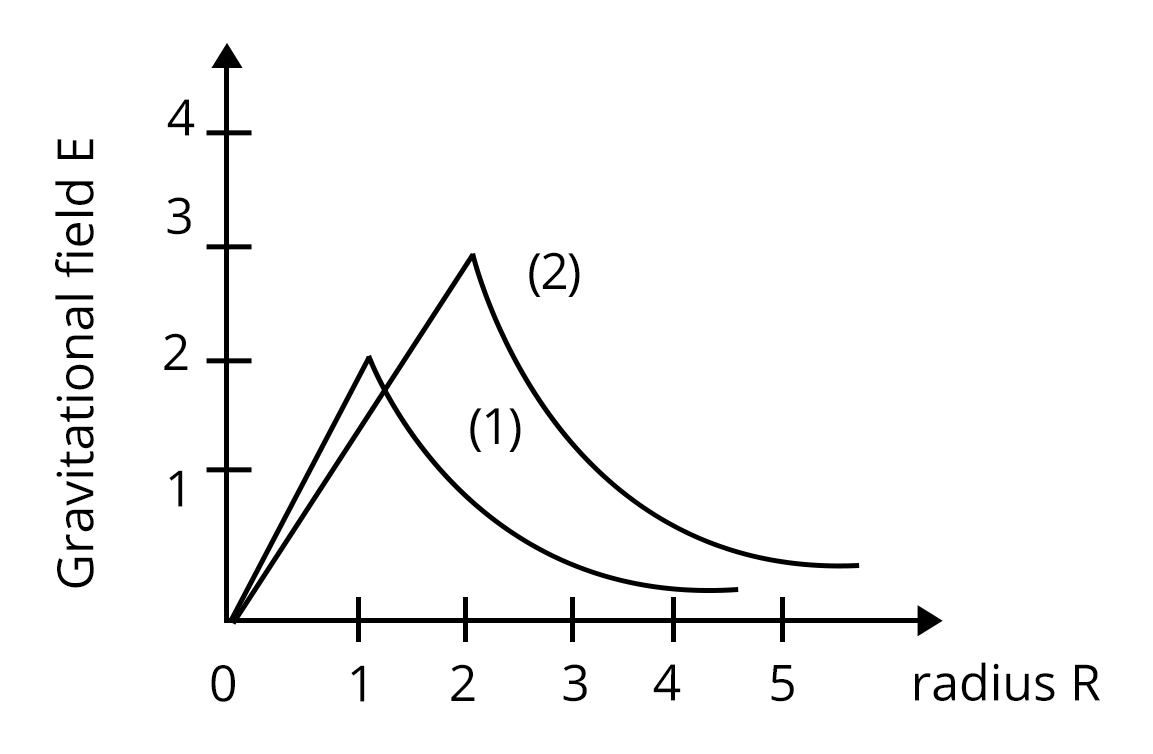Courses
Courses for Kids
Free study material
Free LIVE classes
More

# NEET Important Chapter - Gravitation

Get plus subscription and access unlimited live and recorded courses## Gravitational Force and Newton’s Law of Gravitation: An Important Topic of NEET

Last updated date: 02nd Oct 2023
Total views: 148.5k
Views today: 1.52k

The gravitation chapter covers the most important concepts for NEET, JEE and other competitive exams. In the Gravitation chapter, students will study the basic gravitation definition and Newton's law of gravitation.

The chapter on gravitation begins with the very basic definition of gravity, what is gravitation and Newton’s law of gravitation. Further, the chapter covers the concept of acceleration due to gravity and its variations with different parameters. These concepts will lead us to a very important part of the chapter i.e., planetary motion and Kepler's law. In this chapter, we will get to study many interesting and most important concepts such as gravitational constant, gravitational potential, satellites and terms related to satellites, weightlessness and Binding energy.

The concept of the gravitational field will help us in studying the gravitational potential and potential energy where we can see the resemblance with the electrostatic fields. In this article, we will solve many important numerical problems that will help us in acing the exams.

In this article, we will cover the important concepts and topics that will help students to revise and boost their preparations for NEET/JEE.

### Important Topics of Gravitation

• Universal law of gravitation

• Acceleration due to gravity

• Variations of acceleration due to gravity

• Kepler’s laws of planetary motion

• Gravitation potential and gravitational potential energy

• Satellites

• Binding energy

• Weightlessness

### Important Concepts of Gravitation

 Sl.No Name of the Concept Key Points 1. Universal Law of Gravitation This explains that gravitational force is an attractive force. We experience many gravitation examples in our day to day lives.Newton's universal law of gravitation or Newton's gravitational law states, “The force of attraction between two masses will be directly proportional to the product of masses and inversely proportional to the square of the distance between them.” 2. Acceleration due to gravity It is the acceleration of the body which is subjected to free fall. It is denoted by the letter g.It is independent of the size, shape and mass of the body.The value of g on earth is 9.8 m/s2. 3. Kepler’s first law of planetary motion The first law states, “All the planets in our solar system are revolving around an elliptical orbit with the sun at one of the foci”. 4. Kepler’s second law of planetary motion The second law states, “The rate of the total area swept by the area vector of the revolving planet with respect to the Sun per unit time remains unchanged irrespective of the position of the planets on the set elliptical path.”I.e., $\dfrac {d \vec {A}}{dt}=Constant$Kepler's second law follows conservation of angular momentum. (image will be uploaded soon) 5. Kepler’s third law of planetary motion The third law of planetary motion states, “The square of the period of revolution around the sun of a planet is proportional to the cube of the semimajor axis of its orbit-path around the sun”I.e., ${T^3} \propto {R^3}$ 6. Types of satellites Geostationary Satellite: A geostationary satellite is one that circles around the planet in its equatorial plane at the same angular speed and in the same direction as the earth spins around its own axis.Polar satellite: A polar satellite is a satellite that circles in a polar orbit.Natural Satellite: A natural satellite is a celestial body that circles around a planet. The moon, for example, is a natural satellite of the earth.(image will be uploaded soon) 7. Weightlessness The sensation of weightlessness occurs when the body is unsupported and no external force is acting on it.Because there is no external force acting on an object in free fall with an acceleration equal to that of the earth, it is said to be weightless.For example, when we are travelling through a lift we experience weightlessness.

### List of Important Formulae of Gravitation

 Sl. No Name of the Concept Formulae 1. Newton’s universal law of gravitation $\vec F\propto\dfrac {{m_1}{m_2}}{r^2}$$\vec F=\dfrac {G{m_1}{m_2}}{r^2}Where,G- The universal gravitational constant and its value is 6.67\times {10^{-11}} N{m^2} {kg^{-2}} 2. Acceleration due to gravity of earth g=\dfrac {G {M_e}}{{R_e}^2}Where,Me- The mass of the earthRe -The radius of the earth3. Variation of acceleration due to gravity with height (h) {g_h}=g \left (1- \dfrac {2h}{R_e} \right )4. Variation of acceleration due to gravity with depth (d) {g_d}=g \left (1- \dfrac {d}{R_e} \right ) 5. Variation of acceleration due to gravity with rotation of the earth about its axis {g_{\theta}}=g-{R_e}{\omega^2} \cos^2 \thetaNote:a. At the equator,\theta={0^o}, value acceleration due to gravity {g_{\theta}}=g-{R_e}{\omega^2}b. At the pole, \theta={90^o}, value acceleration due to gravity {g_{\theta}}=g 6. Gravitational field and potential energy E=\dfrac {GM}{R^2}$$U=-\dfrac {GMm}{r}$ 7. Escape velocity of satellites ${V_e}=\sqrt {\dfrac {2GM}{R}}$ 8. Orbital velocity of satellites ${V_o}=\sqrt {\dfrac {GM}{{R_e}+h}}={R_e}\sqrt{\dfrac {g}{{R_e}+h}}$When the satellite is orbiting close to the earth's surface, h << Re, then:${V_o}=\sqrt {g{R_e}}$ 9. Time period of satellite $T=\dfrac {2 \pi}{V_o}$ 10. Total energy of the satellite $E=-\dfrac {G{M_e}m} {2({R_e}+h)}$ 11. Binding Energy $E=-\dfrac {GMm}{2R}$

### Solved Examples of Gravitation

1. An artificial satellite was moving in a circular orbit such that its total energy was 2Eo. Then, calculate the potential energy of the given satellite.

1. E0

2. 3 E0

3. 4 E0

4. -E0

Sol:

Given,

The total energy of the satellite=E=2 Eo

We know that the total energy of the satellite is given by=$E=-\dfrac {G{M_e}m} {2({R_e}+h)}$

Since, Re>>h, we get:

$E=-\dfrac {G{M_e}m} {2{R_e}}$

$E=-\dfrac {G{M_e}m} {2{R_e}}=2 {E_o}$

Now, the potential energy of the satellite is given by:

$U=-\dfrac {G{M_e}m}{R}=2 E=4 {E_o}$

Therefore, the potential energy of the satellite is 4 Eo. Hence, the right option is “c”.

Key Point: The relationship between the total energy and potential energy of the satellite is U=2E.

2. The height at which the acceleration due to gravity becomes $\dfrac {g}{5}$ in terms the radius of the earth R, is

1. $\dfrac {R}{3}$

2. 2R

3. $\dfrac {R}{4}$

4. $\dfrac {2R} {5}$

Sol:

Given,

Acceleration due to gravity at a height “h”=gh=$\dfrac {g}{5}$

Now, we are asked to determine the height h in terms of radius of earth. We know that, variation of acceleration due to gravity with height is given by:

${g_h}=g \left (1- \dfrac {2h}{R_e} \right )$

$\dfrac {g}{5}=g \left (1- \dfrac {2h}{R} \right )$

$h=\dfrac {2R} {5}$

Therefore, option “d” is the right answer.

Key point: Here, the formula of acceleration due to gravity with the variation of height can be used directly.

### Previous Year Questions of Gravitation

1. Two stars of masses m and 2m at a distance d rotate about their common center of mass in free space. The period of revolution is: (JEE 2021)

1. $2 \pi \sqrt {\dfrac {d^3}{3Gm}}$

2. $\dfrac{1} {2 \pi} \sqrt {\dfrac {3Gm}{d^3}}$

3. $\dfrac{1} {2 \pi} \sqrt {\dfrac {d^3}{3Gm}}$

4. ${2 \pi} \sqrt {\dfrac {3Gm}{d^3}}$

Sol:

Given,

Two stars of masses m and 2m are placed at a distance of d. Now, it is being said that they are rotating about their own axis in free space. We know that these two stars are able to rotate in a circular motion due to the force of attraction (gravitational attraction). Then we need to determine the center of mass for these two distances.

We know that, center of mass can be calculated by using below equation:

$C.O.M=\dfrac {{m_1}{x_1}+{m_2}{x_2}} {{m_1}+{m_2}}$

Let us assume that we are considering m as the initial position of the system then we get:

$C.O.M=\dfrac {{m}{(0)}+{2m}{d}} {{m}+{2m}}$

$C.O.M=\dfrac {2d}{3}$

So, now the force due to gravity acting between the two stars will be equal to the centripetal force acting towards the C.O.M. We write:

$\dfrac {Gm(2m)}{d^2}= m \left (\dfrac {2d}{3} \right ){\omega^2}$

${\omega^2}=\dfrac {3Gm}{d^3}$

$T=2\pi \sqrt {\dfrac {d^3}{3Gm}}$

Therefore, option A is the right answer.

Trick: The C.O.M is considered as a static point, thus the masses will always rotate about the same axis.

2. Consider two solid spheres of radii R1 = 1m, R2 = 2m and masses M1 and M2, respectively. The gravitational field due to sphere (1) and (2) are shown. The value of $\dfrac {M_1}{M_2}$ is : (JEE main 2020)1. $\dfrac {2}{3}$

2. $\dfrac {1}{6}$

3. $\dfrac {1}{2}$

4. $\dfrac {1}{3}$

Sol:

Given,

The radii of the two spheres are R1 = 1m, R2 = 2m and masses M1 and M2, respectively. We are asked to determine the ratio of the masses. From the gravitational field equation, we know that:

$E=\dfrac {GM}{R^2}$

For given two spheres we get:

${E_1}=\dfrac {G{M_1}}{{R_1}^2}$

${E_2}=\dfrac {G{M_2}}{{R_2}^2}$

The ratio of masses will be:

$\dfrac {E_1}{E_2}=\dfrac {{M_1}{R_2}^2}{{M_2}{R_1}^2}$

$\dfrac {2}{3}=\dfrac {2^2}{1^2} \dfrac {M_1}{M_2}$

$\dfrac {M_1}{M_2}=\dfrac {1}{6}$

Therefore, the right answer is option b.

Trick: We used the graphs to know the value of gravitational fields instead of using the formula for determining the value of the gravitational field.

### Practice Questions

1. A straight rod of length L extends from x = a to x = L + a. The gravitational force it exerts on a point mass 'm' at x = 0, if the mass per unit length of the rod is A + Bx2, is given by?

Ans: $GM\left [A\left ( \dfrac {1}{a}-\dfrac{1}{a+L}\right )+BL \right ]$

2. If the Earth has no rotational motion, the weight of a person on the equator is W. Determine the speed with which the earth would have to rotate about its axis so that the person at the equator will weigh $\dfrac {3}{4}$ W. Radius of the Earth is 6400 km and g=10 m/s2.

Ans: $0.63 \times {10^-3} rad/sec$

### Conclusion

We conclude that the chapter gravitation has many important concepts that will help us in scoring better marks in NEET and JEE. In this article, we covered gravitation introduction, principles of gravity and all other important concepts from the chapter along with solved and previous year questions. Students can test their knowledge with the help of practice questions.

See More## NEET Important Dates

View All Dates
NEET 2023 exam date and revised schedule have been announced by the NTA. NEET 2023 will now be conducted on 7-May-2023, and the exam registration closes on last week of Dec 2022. You can check the complete schedule on our site. Furthermore, you can check NEET 2023 dates for application, admit card, exam, answer key, result, counselling, etc along with other relevant information.
See More
View All Dates## NEET Information

Application Form
Eligibility Criteria
Reservation Policy
NTA has announced the NEET 2023 application form release date on the official website https://neet.nta.nic.in/. NEET 2023 Application Form is available on the official website for online registration. Besides NEET 2023 application form release date, learn about the application process, steps to fill the form, how to submit, exam date sheet etc online. Check our website for more details.## NEET 2023 Study Material

View NEET Syllabus in Detail
View NEET Syllabus in Detail## NEET 2023 Study Material

View all study material for NEET
All
Physics
Chemistry
Biology
See All## NEET Question PapersView all NEET Important Books
Biology
NCERT Book for Class 12 Biology
Physics
NCERT Book for Class 12 Physics
Chemistry
NCERT Book for Class 12 Chemistry
Physics
H. C. Verma Solutions
See All## NEET Mock Tests

View all mock tests
NEET 2023 free online mock test series for exam preparation are available on the Vedantu website for free download. Practising these mock test papers of Physics, Chemistry and Biology prepared by expert teachers at Vedantu will help you to boost your confidence to face the NEET 2023 examination without any worries. The NEET test series for Physics, Chemistry and Biology that is based on the latest syllabus of NEET and also the Previous Year Question Papers.
See More## NEET 2023 Cut Off

NEET Cut Off
NTA is responsible for the release of the NEET 2023 cut off score. The qualifying percentile score might remain the same for different categories. According to the latest trends, the expected cut off mark for NEET 2023 is 50% for general category candidates, 45% for physically challenged candidates, and 40% for candidates from reserved categories. For the general category, NEET qualifying marks for 2022 ranged from 715-117 general category, while for OBC/SC/ST categories, they ranged from 116-93 for OBC, 116-93 for SC and 116-93 for ST category.
See More## NEET 2023 Results

The NEET 2023 result will be published by NTA on https://neet.nta.nic.in/ in the form of a scorecard. The scorecard will include the roll number, application number, candidate's personal details, and the percentile, marks, and rank of the candidate. Only those candidates who achieve the NEET cut-off will be considered qualified for the exam.
See More
Rank List
Counselling
Cutoff
NEET 2023 state rank lists will be released by the state counselling committees for admissions to the {state-quota-percentage} state quota and to all seats in private medical and dental colleges. NEET 2023 state rank lists are based on the marks obtained in entrance exams. Candidates can check the NEET 2023 state rank list on the official website or on our site.## NEET Top Colleges

View all NEET 2023 Top Colleges
Want to know which Engineering colleges in India accept the NEET 2023 scores for admission to Engineering? Find the list of Engineering colleges accepting NEET scores in India, compiled by Vedantu. There are 1622 Colleges that are accepting NEET. Also find more details on Fees, Ranking, Admission, and Placement.
See More## FAQs on NEET Important Chapter - Gravitation

FAQ

1. Is Gravitation important for the JEE/NEET exam?

Yes, the gravitation chapter is one of the important chapters for JEE and NEET exams. It carries around 1-2 questions in JEE which carry around 4 marks. Due to the formula related questions from this chapter it becomes easy to solve and secure marks in the exams.

2. Is the gravitation chapter tough?

No, the gravitation chapter is simple and easy to understand. The gravitation chapter includes the basic concepts that we got introduced to in our elementary school. If you understand the concepts discussed in this chapter, and practice related questions then you can successfully attempt the questions asked in this chapter in both NEET and JEE exams.

3. Can I crack NEET by solving the previous years’ questions?

According to experts, applicants who practice previous year's papers for JEE and NEET have a good probability of passing the exam. Anyway, practice is the key to success. Students must focus on solving methods and study conceptually along with the PYQ’s.## Notice board

NEET News
NEET BlogsTrending pages